Select Page

# Turing Machine For a^Nb^Nc^N

Design a Turing Machine which recognizes the language L = aNbNcN where N>0.

Given language (L = aNbNcN) will generate equal number of a’s, b’s and c’s. So, consider a input-tap which hold L=”aabbcc” as given below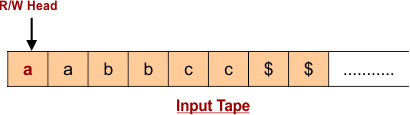## Algorithm For Language aNbNcN

Algorithm for above language in Turing Machine to Accept is given below

• Step 01: Change “a” to “X”
• Step 02: Move Right to First “b”. If “b” found then Change “b” to “Y” otherwise simply reject the language.
• Step 03: Move Right to First “c”. If “c” found then Change “c” to “Z” otherwise simply reject the language.
• Step 04: Move Left to Leftmost “a”
• Step 05: Repeat Step 01 to 04 until no more “a”, “b” and “c” remain in the input tape.

Let construct Turing Machine for Language “aabbcc”.

According to Step 01, Change “a” to “X”.

• State change from q0 to q1

Input tap and Turing Machine behavior is given below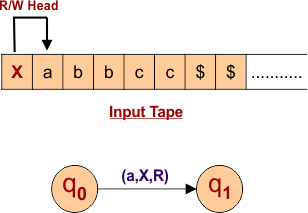According to Step 02, change first right “b” to “Y”.

• State change from q1 to q2

Input tap and Turing Machine behavior is given below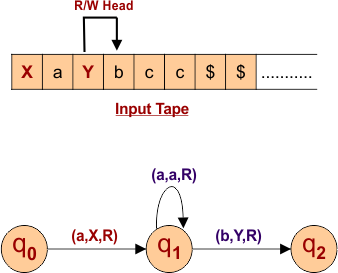According to Step 03, change first right “c” to “Z”.

• State change from q2 to q3

Input tap and Turing Machine behavior is given below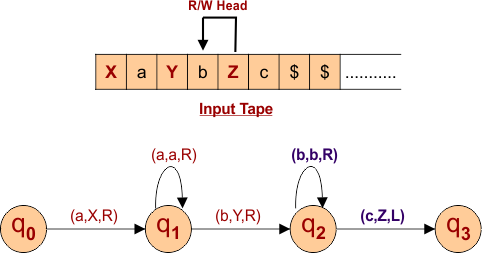Now repeat the step 01 to 04 to replace all “a”, “b” and “c” with “X”, “Y” and “Z” respectively.

According to Step 04 Move Left to Leftmost “a”, Input tap and Turing Machine behavior is given below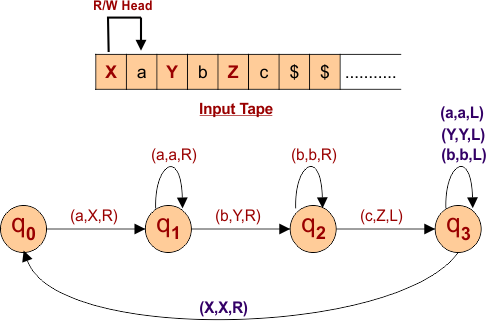Again According to Step 01, replaces leftmost “a” with “X”.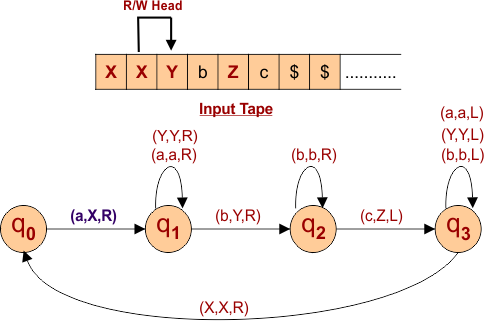Again According to Step 02, replaces first right “b” with “Y”.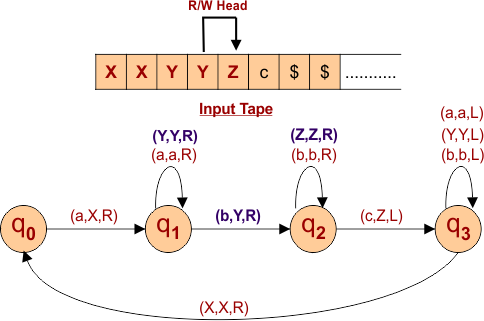According to Step 03, change first right “c” to “Z”. Input tap and Turing Machine behavior is given below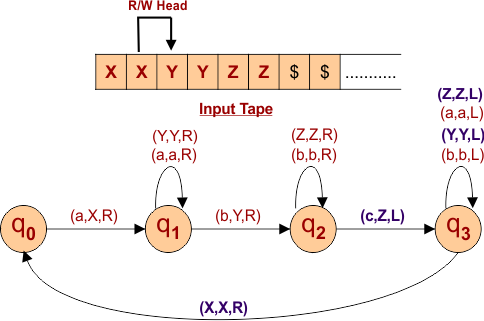At this point all “a”, “b” and “c” are replaced with “X”, “Y” and “Z” respectively.

As the Read/Write Head is pointing X at q0 and Moving toward right. So, to reach at special symbol “\$” consume all “X”, “Y” and “Z”. As below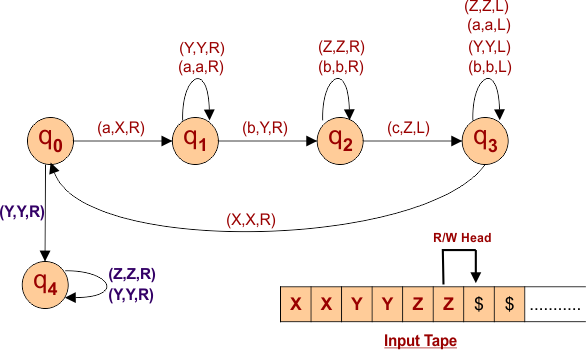Final, by using special symbol “\$”, the R/W head moves toward the last symbol of given language as given below and language is accepted.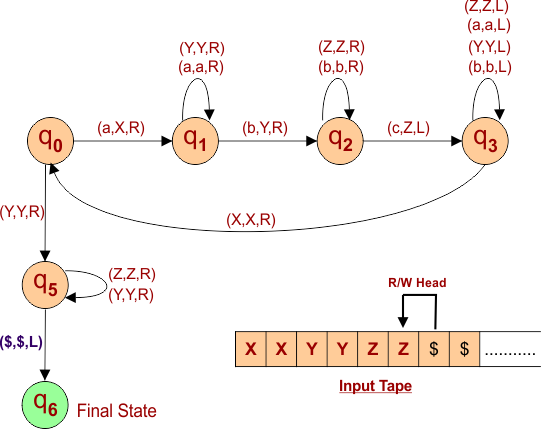Help Other’s By Sharing…